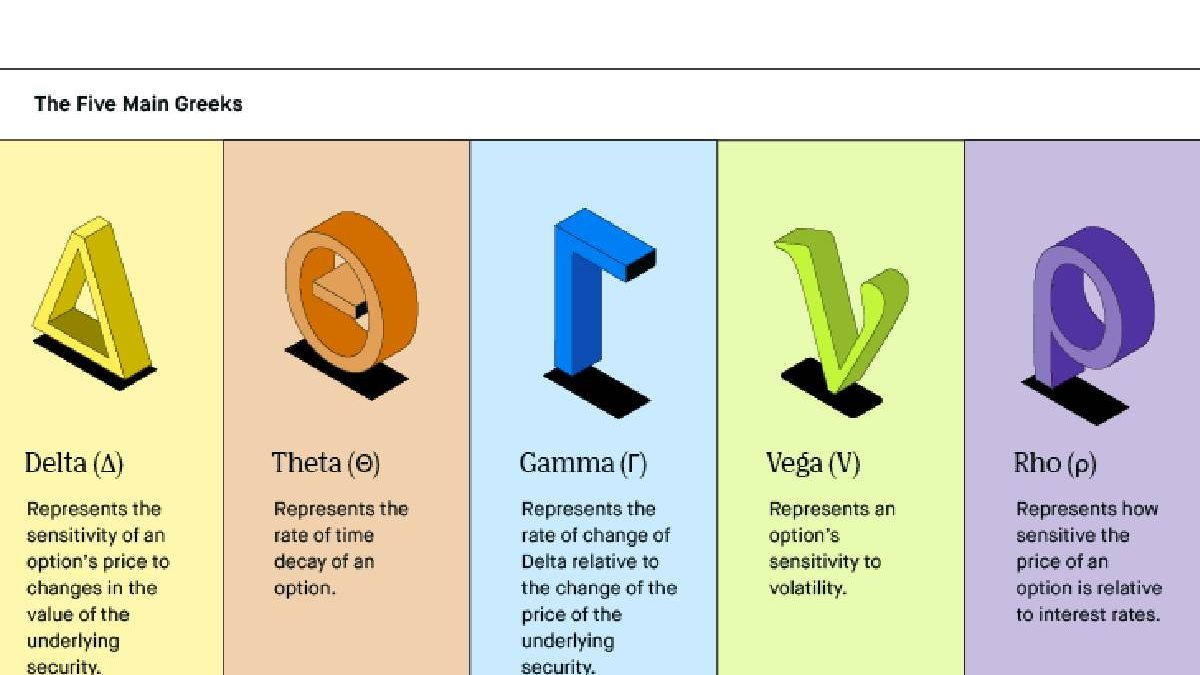07 Dec 2023

# What are delta, gamma, vega, and theta in options trading?Delta, gamma, vega, and theta are four important options trading concepts. Here’s a brief overview of each:

## Delta

Delta measures an option’s price change given a one-point move in the underlying asset’s price.

It’s often referred to as a ‘hedge ratio’ because it can determine how many shares of the underlying asset are needed to hedge (offset) the position. For example, if you have long (own) 100 shares of a certain stock, and the stock’s delta is .50, you would need to sell 50 puts to hedge your position fully.

## Gamma

Gamma is important because it measures the rate of change in delta. When gamma is positive, the delta will increase as the price of the underlying asset increases. It is desirable for long positions because the position will become more ‘delta neutral’ (not as susceptible to changes in the underlying asset’s price) as the price goes up.

Conversely, when gamma is negative, the delta will decrease as the price of the underlying asset increases. It is undesirable for long positions because the position will become more ‘delta hedged’ (more susceptible to changes in the underlying asset’s cost) as the price goes up.

## Vega

Vega is a measure of an option’s sensitivity to changes in implied volatility.

Positive vega means that the option’s price will increase as implied volatility increases, and negative vega means that the option’s price will decrease as implied volatility increases.

## Theta

Theta measures an option’s sensitivity to changes in time to expiration. All else being equal, options with a shorter time to expiration will have higher theta than options with a longer time to expiration. There is less time for the underlying asset’s price to move enough to offset the effects of time decay.

Options with a longer time to expiration will have lower theta because there is more time for the underlying asset’s price to move enough to offset the effects of time decay.

## What are these concepts used for?

Delta, gamma, vega, and theta are essential concepts for options traders because they can be used to determine the best time to enter or exit a trade.

### Use of delta

Delta can be used to assess the risk of a trade. For example, if an option has a high delta, the option is more likely to move in tandem with the underlying asset’s price.

### Use of gamma

Gamma can also be desirable for long positions but may be less so for short positions. If an option has a high gamma, the option’s delta will change rapidly as the underlying asset’s price changes. It will be desirable then for long positions if the underlying asset’s price is expect to increase.

However, suppose the underlying asset’s price is expect to decrease. A high gamma may be undesirable because the position will become more ‘delta hedged’ (more susceptible to changes in the underlying asset’s price).

### Use of vega

If an option has a high vega, the option’s price is sensitive to changes in implied volatility. It may be desirable if the trader expects implied volatility to increase. However, if the trader expects implied volatility to decrease, a high vega may be undesirable because it means that the option’s price will decrease as well.

### Use of theta

Lastly, if an option has a high theta, the option’s price is sensitive to changes in time to expiration. It may be desirable if the trader expects the underlying asset’s price to move quickly. However, if the trader expects the underlying asset’s price to move slowly, a high theta may be undesirable because it means that the option will lose value over time.

## In conclusion

Delta, gamma, vega, and theta are essential concepts for options traders. Because they can be use to determine the best time to enter or exit a trade. These concepts can also be use to assess the risk of a trade. Follow this link if you want to know how to trade options UK.